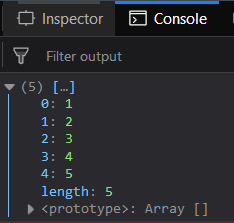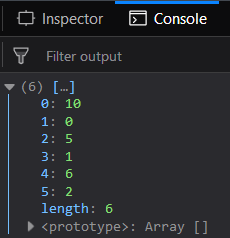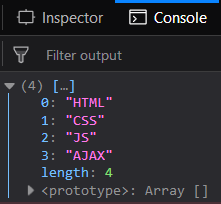# Underscore.js _.uniq() Function

The Underscore.js is a JavaScript library that provides a lot of useful functions like the map, filter, invoke, etc even without using any built-in objects. The _.uniq() function returns the array which does not contain duplicate elements. The first occurrence of the element is included in the resultant array. The operation of checking whether the array is duplicate or not. It is done by the ‘===’ operation.

Syntax:

`_.uniq( array, [isSorted], [iterate] )`

Parameters: This function accepts three parameters which are listed below:

• array: This parameter is used to hold the array of elements.
• isSorted: It is an optional parameter. This parameter is used to hold true for sorted arrays.
• iterate: It is an optional parameter that is used to hold the iterate function.

Return value: It returns an array of unique elements.

Passing a list of numbers to _.uniq() function: The ._uniq() function takes the element from the list one by one and checks whether it is in the resultant array (which is initially empty) by the ‘===’ operator. If it is present then it ignores it and checks the next element. Otherwise, since it is the first occurrence of the element so it is included in the resultant array.

Example:

## html

 `` `<``html``>` `    ``<``head``>` `        ``<``script` `src = ` `"https://cdnjs.cloudflare.com/ajax/libs/underscore.js/1.9.1/underscore-min.js" >` `        ``` `    ``` `    ``<``body``>` `        ``<``script` `type="text/javascript">` `            ``console.log(_.uniq([1, 2, 3, 4, 5, 4, 3, 2, 1]));` `        ``` `    ``` ``

Output:Passing the second parameter as false to the _.uniq() function: If pass the second parameter as false along with the array then the _.uniq() function will work in a similar manner as in the first example. All the unique elements will be present in the resultant array.

Example:

## html

 `` `<``html``>` `    ``<``head``>` `        ``<``script` `src = ` `"https://cdnjs.cloudflare.com/ajax/libs/underscore.js/1.9.1/underscore-min.js" >` `        ``` `    ``` `    ``<``body``>` `        ``<``script` `type="text/javascript">` `            ``console.log(_.uniq([10, 0, 5, 1, 6, 10, 2, 1, 2], false));` `        ``` `    ``` ``

Output:Passing the second parameter as true to the _.uniq() function: If pass the second parameter as true along with the array then the _.uniq() function will not work in a similar manner rather it will perform any operation on the array. Hence, the resultant array will contain all the elements of the array passed in the same order in which it appeared in the passed array.

Example:

## html

 `` `<``html``>` `    ``<``head``>` `        ``<``script` `src = ` `"https://cdnjs.cloudflare.com/ajax/libs/underscore.js/1.9.1/underscore-min.js" >` `        ``` `    ``` `    ``<``body``>` `        ``<``script` `type="text/javascript">` `            ``console.log(_.uniq([10, 0, 5, 1, 6, 10, 2, 1, 2], true));` `        ``` `    ``` ``

Output:Passing words to the _.uniq() function: If passing the set of strings to the _.uniq() function then it will work in a similar manner as it will work with numbers etc. Therefore, the resultant array will contain only the first occurrence of all the repeated elements in the resultant array.

Example:

## html

 `` `<``html``>` `    ``<``head``>` `        ``<``script` `src = ` `"https://cdnjs.cloudflare.com/ajax/libs/underscore.js/1.9.1/underscore-min.js" >` `        ``` `    ``` `    ``<``body``>` `        ``<``script` `type="text/javascript">` `            ``console.log(_.uniq(["HTML", "CSS", "JS",` `                        ``"AJAX", "CSS", "JS", "CSS"]));` `        ``` `    ``` ``

Output:`<``script` `type="text/javascript" src = ` `"https://cdnjs.cloudflare.com/ajax/libs/underscore.js/1.9.1/underscore-min.js"> ` ``